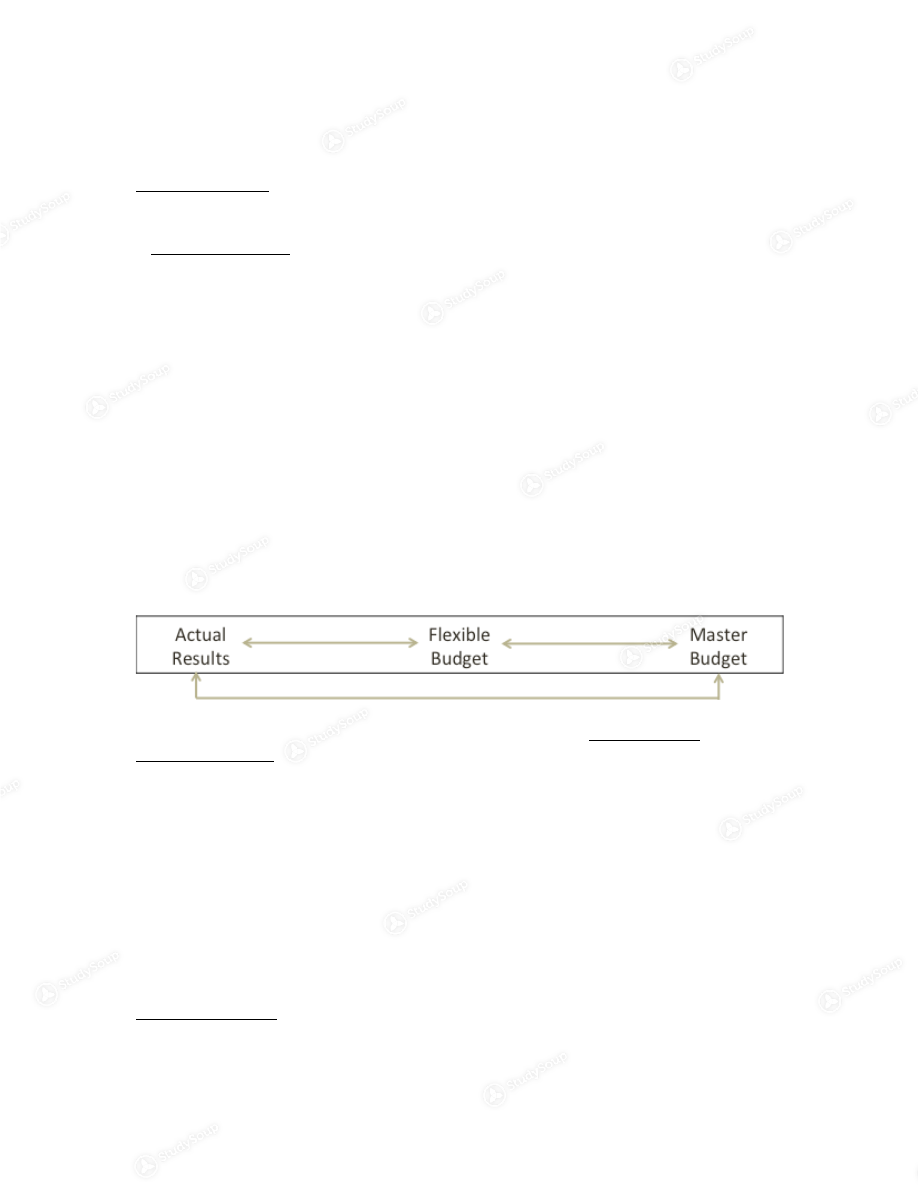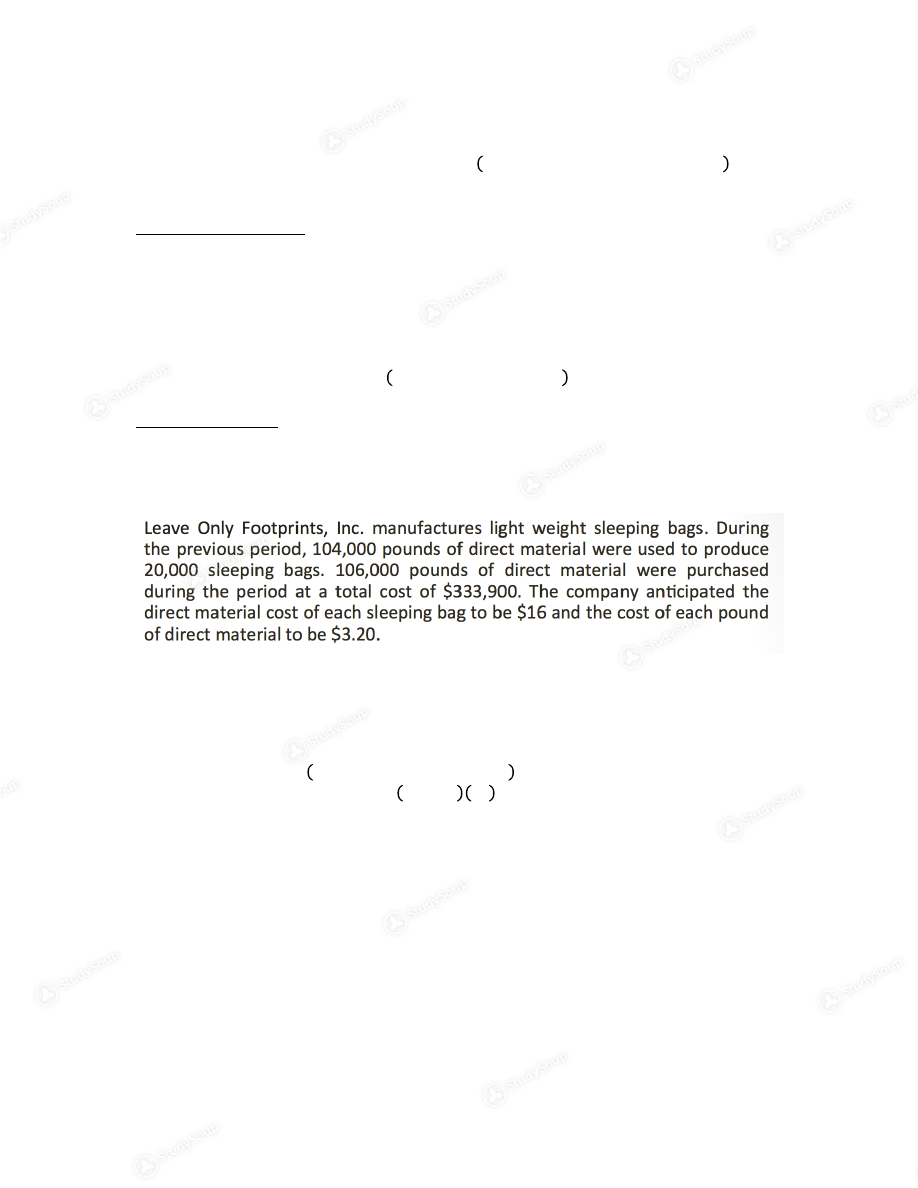×

# UGA - ACCT 2102 - Class Notes - Chapter 11 Notes

### Created by: Rachel Moore Elite Notetaker

Schools > University of Georgia > Accounting > ACCT 2102 > UGA - ACCT 2102 - Class Notes - Chapter 11 Notes

UGA - ACCT 2102 - Class Notes - Chapter 11 Notes

0 5 3 53 Reviews
This preview shows pages 1 - 2 of a 4 page document. to view the rest of the contentCreated By: Rachel Moore    Not for redistribution.  ACCT 2102 April 11, 2016 – April 15, 2016 Chapter 11 Notes Standard Costs and Variances

A standard unit cost is a budget for a singe unit of product.

Direct Material
•  𝑆𝑡𝑎𝑛𝑑𝑎𝑟𝑑 𝐷𝑀 𝑐𝑜𝑠𝑡 𝑝𝑒𝑟 𝑢𝑛𝑖𝑡 = 𝑠𝑡𝑎𝑛𝑑𝑎𝑟𝑑 𝑞𝑢𝑎𝑛𝑡𝑖𝑡𝑦 𝑜𝑓 𝐷𝑀 × 𝑠𝑡𝑎𝑛𝑑𝑎𝑟𝑑 𝑝𝑟𝑖𝑐𝑒 𝑜𝑓 𝐷𝑀 •  2 pounds of DM and DM is \$5 per pound ! standard DM unit cost = \$10
Direct Labor
•  𝑆𝑡𝑎𝑛𝑑𝑎𝑟𝑑 𝐷𝐿 𝑐𝑜𝑠𝑡 𝑝𝑒𝑟 𝑢𝑛𝑖𝑡  =  𝑠𝑡𝑎𝑛𝑑𝑎𝑟𝑑 𝑞𝑢𝑎𝑛𝑡𝑖𝑡𝑦 𝑜𝑓 𝐷𝐿 × 𝑠𝑡𝑎𝑛𝑑𝑎𝑟𝑑 𝑟𝑎𝑡𝑒 𝑜𝑓 𝐷𝐿  •  5 hours of DL and DL wage rate is \$10/hour ! standard SL unit cost = \$50
•  𝑆𝑡𝑎𝑛𝑑𝑎𝑟𝑑 𝑀𝑂𝐻 𝑐𝑜𝑠𝑡 𝑝𝑒𝑟 𝑢𝑛𝑖𝑡  =  𝑠𝑡𝑎𝑛𝑑𝑎𝑟𝑑 𝑞𝑢𝑎𝑛𝑡𝑖𝑡𝑦 𝑜𝑓 𝑐𝑜𝑠𝑡 𝑑𝑟𝑖𝑣𝑒𝑟 × 𝑃𝑂𝐻𝑅  •  5 hours of DL and POHR of \$8 per DL hours ! standard MOH unit cost = \$40
Using examples above, determine the standard unit cost… \$10 + \$50 + \$40 = \$100
**The standard quantity is als referred to as the input ratio.** The Flexible Budget Variance can be further divided into a Price Variance and a Quantity Variance. •  Price/Rate Variance – the difference betweem what we paid and what we expected to pay •  Quantity/Efficiency variance – the difference between what we used and expected to use for out actual output
In this chapter, (+) and (-) signs are important with variances. A positive variance
is an unfavorable variance. A negative variance is a favorable variance. Variances
will always be calculated as 𝐴𝐶𝑇𝑈𝐴𝐿 –  𝐵𝑈𝐷𝐺𝐸𝑇.
Direct Material Variances
DM Price Variance
•  Difference between what we paid and what we expected to pay for DM
•  Can first be calculated at point of purchaseCreated By: Rachel Moore    Not for redistribution.  •  Responsible party is purchasing manager 𝐷𝑀 𝑝𝑟𝑖𝑐𝑒  =  𝑞𝑢𝑎𝑛𝑡𝑖𝑡𝑦 𝑝𝑢𝑟𝑐ℎ𝑎𝑠𝑒𝑑 ×  𝑎𝑐𝑡𝑢𝑎𝑙 𝑝𝑟𝑖𝑐𝑒 –  𝑠𝑡𝑎𝑛𝑑𝑎𝑟𝑑 𝑝𝑟𝑖𝑐𝑒  𝐷𝑀 𝑝𝑟𝑖𝑐𝑒  =   (𝑞 𝑝𝑢𝑟𝑐ℎ)(𝑎𝑐𝑡𝑢𝑎𝑙 \$) – (𝑞 𝑝𝑢𝑟𝑐ℎ)(𝑠𝑡𝑎𝑛𝑑𝑎𝑟𝑑 \$)   DM Quantity Variance •  Difference between the quantity used and the quantity expected for actual output •  Can first be calculated at point of production
•  Responsible party is production manager
𝐷𝑀 𝑞𝑢𝑎𝑛𝑡𝑖𝑡𝑦  =  𝑠𝑡𝑎𝑛𝑑𝑎𝑟𝑑 𝑝𝑟𝑖𝑐𝑒 × (𝑞𝑢𝑎𝑛𝑡𝑖𝑡𝑦 𝑢𝑠𝑒𝑑 –  𝑞𝑢𝑎𝑛𝑡𝑖𝑡𝑦 𝑒𝑥𝑝𝑒𝑐𝑡𝑒𝑑)  𝑄𝑢𝑎𝑛𝑡𝑖𝑡𝑦 𝑒𝑥𝑝𝑒𝑐𝑡𝑒𝑑  =   𝑠𝑡𝑎𝑛𝑑𝑎𝑟𝑑 𝑖𝑛𝑝𝑢𝑡 𝑟𝑎𝑡𝑖𝑜  × (𝑎𝑐𝑡𝑢𝑎𝑙 𝑜𝑢𝑡𝑝𝑢𝑡)
Total DM Variance
𝑇𝑜𝑡𝑎𝑙 𝐷𝑀 𝑉𝑎𝑟𝑖𝑎𝑛𝑐𝑒 =  𝐴𝑐𝑡𝑢𝑎𝑙 –  𝐵𝑢𝑑𝑔𝑒𝑡 𝑇𝑜𝑡𝑎𝑙 𝐷𝑀 𝑉𝑎𝑟. =   (𝑞𝑢𝑎𝑛𝑡𝑖𝑡𝑦 𝑢𝑠𝑒𝑑)(𝑎𝑐𝑡𝑢𝑎𝑙 \$) – (𝑞𝑢𝑎𝑛𝑡𝑖𝑡𝑦 𝑒𝑥𝑝𝑒𝑐𝑡𝑒𝑑)(𝑠𝑡𝑎𝑛𝑑𝑎𝑟𝑑 \$)  𝐷𝑀 𝑝𝑟𝑖𝑐𝑒  =   𝑞𝑡𝑦 𝑝𝑢𝑟𝑐ℎ (𝑎𝑐𝑡 \$  −  𝑠𝑡𝑑 \$)
𝐷𝑀 𝑝𝑟𝑖𝑐𝑒 = 106,000 (\$3.15 − \$3.20)
𝐷𝑀 𝑝𝑟𝑖𝑐𝑒 = < \$5,300 > (𝐹)
𝐷𝑀 𝑞𝑢𝑎𝑛𝑡𝑖𝑡𝑦 = 𝑠𝑡𝑑 \$  𝑞𝑡𝑦 𝑢𝑠𝑒𝑑 − 𝑞𝑡𝑦 𝑒𝑥𝑝𝑒𝑐𝑡𝑒𝑑
𝐷𝑀 𝑞𝑢𝑎𝑛𝑡𝑖𝑡𝑦 = \$3.20 (104,000 – 20,000 5 ) 𝐷𝑀 𝑞𝑢𝑎𝑛𝑡𝑖𝑡𝑦 = \$12,800 (𝑈) *\$16 / \$3.20 = 5 lbs/unit
𝑇𝑜𝑡𝑎𝑙 𝐷𝑀 𝑉𝑎𝑟𝑖𝑎𝑛𝑐𝑒  =   (𝑞𝑡𝑦 𝑢𝑠𝑒𝑑)(𝑎𝑐𝑡 𝑝𝑟𝑖𝑐𝑒) – (𝑞𝑡𝑦 𝑒𝑥𝑝)(𝑠𝑡𝑑 𝑝𝑟𝑖𝑐𝑒)
𝑇𝑜𝑡𝑎𝑙 𝐷𝑀 𝑉𝑎𝑟𝑖𝑎𝑛𝑐𝑒  =   (104,000)(\$3.15) – (100,000)(\$3.20)  𝑇𝑜𝑡𝑎𝑙 𝐷𝑀 𝑉𝑎𝑟𝑖𝑎𝑛𝑐𝑒  =  \$7,600 (𝑈)
How would our calculations change if 106,000 pounds of direct material were used
during the period?
DM quantity would change from \$12,800 (U) to
\$19,200 (U) . This would also change the total DM variance to \$13,900 (U) .

This is the end of the preview. Please to view the rest of the contentJoin more than 18,000+ college students at University of Georgia who use StudySoup to get ahead